• https://www.jianshu.com/p/a20e11416a25 看的三种算法的完整版
https://www.jianshu.com/p/a20e11416a25
看的三种算法的完整版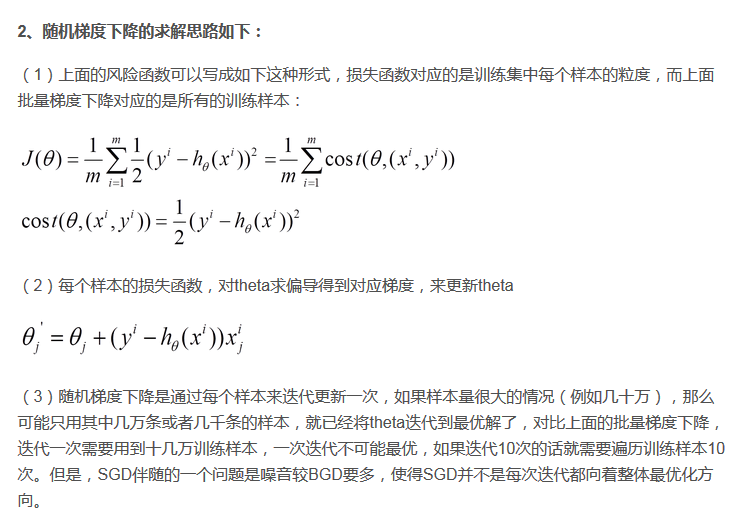展开全文• 在学习线性回归的时候很多课程都会讲到用梯度下降法求解参数，对于梯度下降算法怎么求出这个解讲的较少，自己实现一遍算法比较有助于理解算法，也能注意到比较细节的东西。具体的数学推导可以参照这一篇博客...
      在学习线性回归的时候很多课程都会讲到用梯度下降法求解参数，对于梯度下降算法怎么求出这个解讲的较少，自己实现一遍算法比较有助于理解算法，也能注意到比较细节的东西。具体的数学推导可以参照这一篇博客（http://www.cnblogs.com/pinard/p/5970503.html）
一、       首先，我们用一个简单的二元函数用梯度下降法看下算法收敛的过程
也可以改一下eta，看一下步长如果大一点，算法的收敛过程
import numpy as np
import matplotlib.pyplot as plt

plot_x = np.linspace(-1,6,140)
plot_y = (plot_x-2.5)**2-1

#先算出来当前函数的导数
def dJ(theta):
return 2*(theta-2.5)

#梯度函数
def J(theta):
return (theta-2.5)**2-1

#初始化theta=0
#步长eta设置为0.1
eta = 0.1
theta_history = []
theta = 0
epsilon = 1e-8
while True:
gredient = dJ(theta)
last_theta = theta
theta = theta - eta*gredient
theta_history.append(theta)

if(abs(J(theta) - J(last_theta)) < epsilon):
break

print(theta)
print(J(theta))

plt.plot(plot_x, J(plot_x))
plt.plot(np.array(theta_history),J(np.array(theta_history)),color='r',marker='+')
plt.show()
出来的结果如下：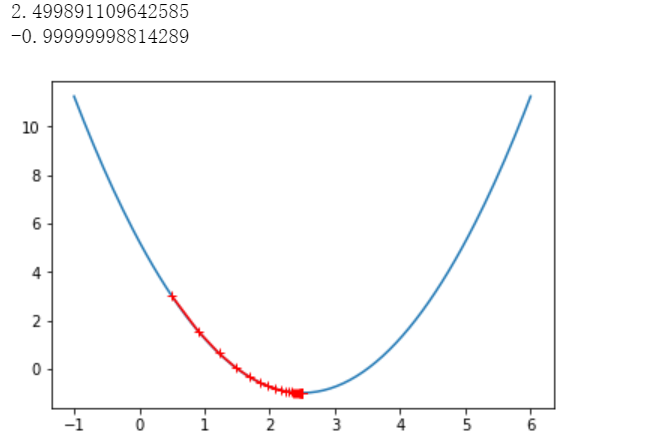首先，构建一个函数
import numpy as np
import matplotlib.pyplot as plt

np.random.seed(666)
x = 2 * np.random.random(size=100)
y = x*3. + 4. + np.random.normal(size=100)

#然后改成向量的形式
X = x.reshape(-1,1)

plt.scatter(x,y)
plt.show()
然后写实现梯度下降法求解我们构建的这个函数：
def J(theta , X_b , y):
try:
return sum((y-X_b.dot(theta))**2)/len(X_b)
except:
return float('inf')

#这里使用的是每次求一个参数，然后组合在了一起成了res
def dJ(theta, X_b ,y):
res = np.empty(len(theta))
res = np.sum(X_b.dot(theta) - y)
for i in range(1, len(theta)):
res[i] = (X_b.dot(theta) - y).dot(X_b[:,i])
return res * 2 / len(X_b)

#这里也可以直接用矩阵运算求出所有的参数，效率更高
#return X_b.T.dot(X_b.dot(theta)-y)*2. / len(y)


然后把上面的过程封装成函数形式：
#把整个算法写成函数的形式

def gradient_descent(X_b, y ,inital_theta, eta ,n_inters = 1e4, epsilon = 1e-8):
theta = initial_theta
i_inter = 0

while i_inter < n_inters:
last_theta = theta

if(abs(J(theta,X_b,y) - J(last_theta,X_b,y)) < epsilon):
break

i_inter += 1
return theta
然后用我们实现的算法求解上面那个函数：
#这里加一列1
X_b = np.hstack([np.ones((len(x),1)), x.reshape(-1,1)])
#初始theta设置为0
initial_theta = np.zeros(X_b.shape)
eta = 0.01

theta = gradient_descent(X_b, y, initial_theta, eta)
theta
输出结果如下：
array([4.02145786, 3.00706277])

使用梯度下降法时，由于不同维度之间的值大小不一，最好将数据进行归一化，否则容易造成不收敛

随机梯度下降法可以训练更少的样本就得到比较好的效果，下面用两段代码比较下。
这个就是之前的批量梯度下降，不过换了一个数据集
import numpy as np
import matplotlib.pyplot as plt

m = 100000

x = np.random.normal(size = m)
X = x.reshape(-1,1)
y = 4. * x + 3. +np.random.normal(0,3,size = m)

def J(theta , X_b , y):
try:
return sum((y-X_b.dot(theta))**2)/len(X_b)
except:
return float('inf')

def dJ(theta, X_b ,y):
return X_b.T.dot(X_b.dot(theta)-y)*2. / len(y)

def gradient_descent(X_b, y ,inital_theta, eta ,n_inters = 1e4, epsilon = 1e-8):
theta = initial_theta
i_inter = 0

while i_inter < n_inters:
last_theta = theta

if(abs(J(theta,X_b,y) - J(last_theta,X_b,y)) < epsilon):
break

i_inter += 1
return theta
%%time
X_b = np.hstack([np.ones((len(x),1)), X])
initial_theta = np.zeros(X_b.shape)
eta = 0.01

theta = gradient_descent(X_b, y, initial_theta, eta)
theta
结果如下：
Wall time: 37.2 s
theta：
array([3.00590902, 4.00776602])
下面我们用随机梯度下降：
#这里每次求一行数据的梯度,所以后面不用除以m
def dJ_sgd(theta, X_b_i, y_i):
return X_b_i.T.dot(X_b_i.dot(theta) - y_i)* 2.

#随机梯度下降法学习率设置t0/(t+t1)这种形式
#由于梯度下降法随机性，设置最后的结果的时候只设置最大迭代次数
def sgd(X_b, y, initial_theta, n_iters):

t0 = 5
t1 = 50

def learning_rate(t):
return t0/(t+t1)

theta = initial_theta
for cur_iter in range(n_iters):
#下面是设置每次随机取一个样本
rand_i = np.random.randint(len(X_b))
theta = theta - learning_rate(cur_iter) * gradient

return theta

%%time
X_b = np.hstack([np.ones((len(x),1)), X])
initial_theta = np.zeros(X_b.shape)

theta = sgd(X_b, y, initial_theta, n_iters=len(X_b)//3)

结果如下：
Wall time: 481 ms
theta:
array([2.93906903, 3.99764075])
对比下两者的运行时间，随机梯度下降法计算量更小，时间也大大减少。

这个完全按照自己理解写下，如果有大牛指点下不胜感激。
小批量梯度下降法主要在于每次训练的数据量不同，随机梯度下降是有一个样本就训练一次，小批量梯度下降是有一批样本训练一次，这里默认参数我给100
#这里每次求一行数据的梯度,所以后面不用除以m
def dJ_sgd(theta, X_b_i, y_i):
return X_b_i.T.dot(X_b_i.dot(theta) - y_i)* 2.

def sgd(X_b, y, initial_theta, n_iters,n=100):

t0 = 5
t1 = 50

def learning_rate(t):
return t0/(t+t1)

theta = initial_theta
for cur_iter in range(n_iters):
#下面是设置每次随机取一个样本
for i in range(n):
rand_i = []
rand_i_1 = np.random.randint(len(X_b))
rand_i.append(rand_i_1)

theta = theta - learning_rate(cur_iter) * gradient
return theta
然后还是用之前的数据集测试下：
%%time
import numpy as np
X_b = np.hstack([np.ones((len(x),1)), X])
initial_theta = np.zeros(X_b.shape)

theta = sgd(X_b, y, initial_theta,n=5, n_iters=len(X_b)//3)
结果如下：
Wall time: 643 ms
这里每次给5个样本，耗费的时间还是很长的，不知道是不是代码写的有问题。
结果来看是对的:
array([2.96785569, 4.00405719])
展开全文• 当用于训练的数据量非常大时，批量梯度下降算法变得不再适用(此时其速度会非常慢)，为解决这个问题，人们又想出了随机梯度下降算法随机梯度下降算法的核心思想并没有变，它仍是基于梯度，通过对目标函数中的参数...
当用于训练的数据量非常大时，批量梯度下降算法变得不再适用(此时其速度会非常慢)，为解决这个问题，人们又想出了随机梯度下降算法。随机梯度下降算法的核心思想并没有变，它仍是基于梯度，通过对目标函数中的参数不断迭代更新，使得目标函数逐渐靠近最小值。
具体代码实现如下：
先导入要用到的各种包：
%matplotlib notebook
import pandas as pd
import matplotlib.pyplot as plt
import numpy as np
读入数据并查看数据的相关信息：
data = pd.read_csv('ex1data1.txt',header = None,names=['Population','Profits'])
data.head() # 查看data中的前五条数据
data中前五条数据如下图所示：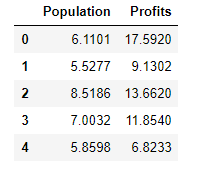data.describe() # 查看data的各描述统计量信息
如下图所示：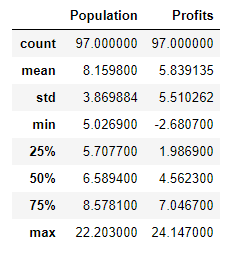绘制原始数据的散点图：
fig,axes = plt.subplots()
data.plot(kind='scatter',x='Population',y='Profits',ax=axes,marker='o',color='r')
axes.set(xlabel='Population',ylabel='Profits')
fig.savefig('p1.png')
绘制的散点图为：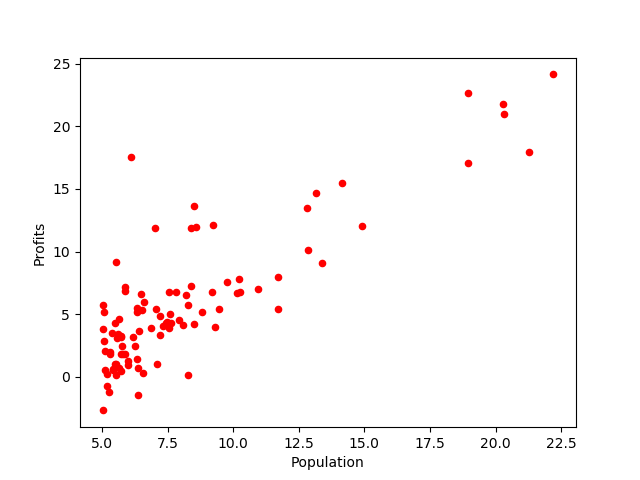向data中添加一列便于矩阵计算的辅助列：
data.insert(0,'Ones',1)
data.head()
加入辅助列的data如下所示：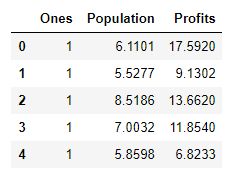随机梯度下降的实现：
# 定义数据特征和标签的提取函数：
def get_fea_lab(data):
cols = data.shape
X = data.iloc[:,0:cols-1] # X是data中的前两列(不包括索引列)
y = data.iloc[:,cols-1:cols] # y是data中的最后一列

# 将X和y都转化成矩阵的形式：
X = np.matrix(X.values)
y = np.matrix(y.values)
return X,y

# 定义代价函数：
def computeCost(data,theta,i):
X,y = get_fea_lab(data)
inner = np.power(((X*theta.T)-y),2)
return (float(inner[i]/2))

# 定义随机梯度下降函数：

X0,y0 = get_fea_lab(data)  # 提取X和y矩阵
temp = np.matrix(np.zeros(theta.shape))
parameters = int(theta.shape)
cost = np.zeros(len(X0))
avg_cost = np.zeros(epoch)

for k in range(epoch):
new_data = data.sample(frac=1) # 打乱数据
X,y = get_fea_lab(new_data)  # 提取新的X和y矩阵

for i in range(len(X)):
error = X[i:i+1]*theta.T-y[i]
cost[i] = computeCost(new_data,theta,i)

for j in range(parameters):
temp[0,j] = theta[0,j] - alpha*error*X[i:i+1,j]

theta = temp
avg_cost[k] = np.average(cost)

return theta,avg_cost

# 初始化学习率、迭代轮次和参数theta：
alpha = 0.001
epoch = 200
theta = np.matrix(np.array([0,0]))

# 调用随机梯度下降函数来计算线性回归中的theat参数：

# g的值为matrix([[-3.77650181,  1.29548466]])
绘制每轮迭代中代价函数的平均值与迭代轮次的关系图像：
本例中因为数据集中一共只有97个样本，所以对于每轮迭代，我选择的是计算所有样本对应的的代价函数的平均值。在数据集非常大的情况下，我们可以选择计算每轮迭代中最后一部分样本对应的代价函数的平均值。
fig, axes = plt.subplots()
axes.plot(np.arange(epoch), avg_cost, 'r')
axes.set_xlabel('Epoch')
axes.set_ylabel('avg_cost')
axes.set_title('avg_cost vs. Epoch')
fig.savefig('p2.png')
具体如下图所示：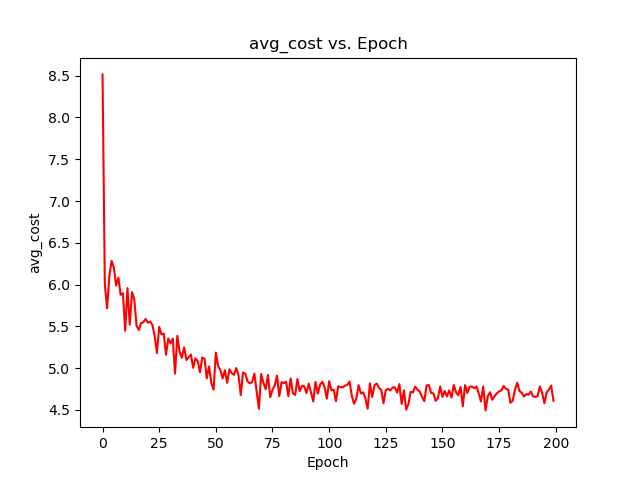从上图中我们可以看到，大约从第90轮迭代开始，代价函数的平均值在某个值上下进行小范围波动(某个值其实就是值全局最小值)。前面，我们把最大迭代轮次设为了200，并据此计算除了线性回归参数theta的值为matrix([[-3.77650181, 1.29548466]])。而用正规方程计算出的theta参数的精确值为matrix([[-3.89578088],[ 1.19303364]])，二者的差别在可接受范围内。关于用正规方程求解线性回归参数可以参考：https://blog.csdn.net/qq_41080850/article/details/85292769、https://blog.csdn.net/qq_41080850/article/details/85159645。
根据前文计算出的theta参数值，绘制原始数据的线性拟合图：
x = np.linspace(data.Population.min(),data.Population.max(),100)
f = g[0,0] + g[0,1]*x

fig,axes = plt.subplots()
axes.plot(x,f,'r',label='Fitted')
axes.scatter(x=data.Population,y=data.Profits,label='Trainning data')
axes.legend(loc='best')
axes.set(xlabel='Population',ylabel='Profits',title='Population vs. Profits')
fig.savefig('p3.png')
绘制的线性拟合图为：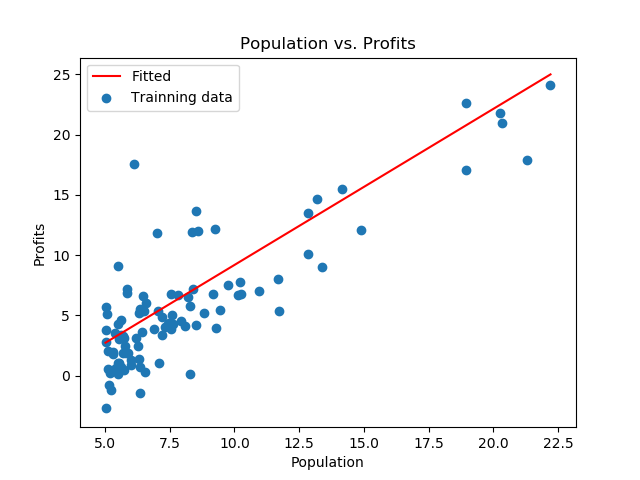批量梯度下降算法与随机梯度下降算法的比较：
1）批量梯度下降算法在每次迭代更新目标函数的参数时，是将训练数据集中的所有样本都考虑进去，以此计算代价函数；而随机梯度下降算法在每次迭代更新目标函数的参数时，只考虑数据集中的一个样本并据此计算代价函数。因此，当训练数据集非常大时，随机梯度下降的迭代速度要比批量梯度下降的迭代速度快很多。
两者的代价函数具体如下图所示：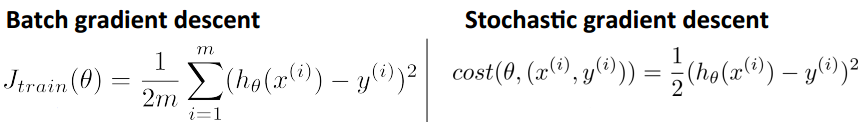上图中，左边是批量梯度下降的代价函数，右边是随机梯度下降的代价函数。
2）不同于批量梯度下降，当迭代到一定轮次时，随机梯度下降计算出的代价函数是在某个靠近全局最小值的区域内徘徊，而不是直接逼近全局最小值并停留在那点。关于随机梯度下降的收敛性，可以参考：https://www.zhihu.com/question/27012077。
其他参考资料：
《Python Machine Learning Second Edition》——Vahid Mirjalili&Sebastian Raschka
Andew Ng机器学习公开课
https://blog.csdn.net/qq_22238533/article/details/70917102
PS：本文为博主原创文章，转载请注明出处。
展开全文• 梯度下降算法 梯度下降，依照所给数据，判断函数，随机给一个初值w，之后通过不断更改，一步步接近原函数的方法。更改的过程也就是根据梯度不断修改w的过程。 以简单的一元函数为例 原始数据为 x_data = [1.0,...
梯度下降算法
梯度下降，依照所给数据，判断函数，随机给一个初值w，之后通过不断更改，一步步接近原函数的方法。更改的过程也就是根据梯度不断修改w的过程。
以简单的一元函数为例
原始数据为
x_data = [1.0,2.0,3.0]
y_data = [2.0,4.0,6.0]
因此我们设置函数为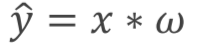对于该函数，我们的w是未知的，因此如何根据xy的数据，获取到正确的w值就是梯度下降的目标。
首先我们要先给定一个随机w值，这个值可以是任何数，我们的算法就会根据我们所计算的cost函数，判断偏离正确数据有多大，之后根据梯度，对w进行更新，直到cost为0，我们也就获取到正确的w值。
cost函数，也就是根据自己的模拟量，算出的结果与原函数所给数据的差值的平方
cost函数的表示为（所求的是N个数据的平均cost）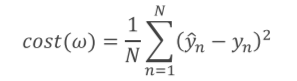cost函数对w的求导为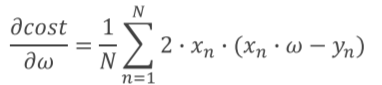每次更改的过程就是不断更新w，（a也就是每次w改变的步长，用a乘以w的偏导）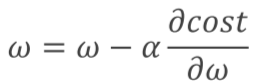最终当cost为0时，就基本可以保证函数模拟的是正确的。
梯度下降的python实现
x_data = [1.0,2.0,3.0]
y_data = [2.0,4.0,6.0]
w = 1.0

def forward(x):
return x*w
def cost(xs,ys):
cost = 0
for x,y in zip(xs,ys):
y_pred = forward(x)
cost+=(y_pred - y) **2
return cost / len(xs)
'''求平均的cost大小'''

for x,y in zip(xs,ys):
'''求平均的梯度大小'''

print("Predict (before training)",4,forward(4))
for epoch in range(100):
cost_val = cost(x_data,y_data)
print('EPOCH:',epoch,'w=',w,'loss=',cost_val)
print("Predict(after training)",4,forward(4))
'''对下一个数据进行预测的结果'''
由于梯度算法，是对所有的差值求平均，因此，很有可能困在局部最优解之中。举个例子，一个人在下山的过程中，不断找周围的最低点，有的人可以直接下山，但是有的人在半山腰山遇到一个水池，对这个水池来说，四周都比它高，因此，就会被困在这个水池中，没法下山。因此解决办法就是随机梯度下降。
随机梯度下降
采用随机梯度下降，相较于求平均的cost，采用随机的loss函数，也就是每次只取一个值，还是上个例子，当这个人困在水池中是，突然随机出现一个点，告诉你你的周围还有更低点，你就可以走出水池，然后重新走向下山的道路
求w的导数函数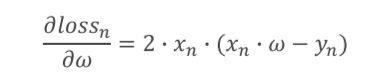loss函数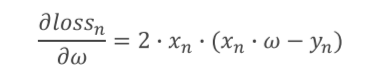对于x，y参数，不像梯度下降的cost函数要遍历x，y的原数据，而只是使用当前的数据x,y即可
随机梯度下降的python实现
x_data = [1.0,2.0,3.0]
y_data = [2.0,4.0,6.0]
w = 1.0

def forward(x):
return x*w

def loss(x,y):
y_pred = forward(x)
return(y_pred-y)**2

return 2*x*y*(x*w-y)

print('Predict(before training)',4,forward(4))
for epoch in range(100):
for x,y in zip(x_data,y_data):
'''相比于梯度下降需要一次对所有数据求取平均值，随机梯度下降需要进行两次循环,
在第二次循环中，对于每个数据都要单独求取一个梯度'''
'''分别对三个数据求取梯度'''
l = loss(x,y)
print("progress:",epoch,"w=",w,"loss",l)
print("Prediect (after training)",4,forward(4))

展开全文• 文章目录线性回归学习目标2.5 梯度下降法介绍1 全梯度下降算法（FG）2 随机梯度下降算法（SG）3 小批量梯度下降算法（mini-bantch）4 随机平均梯度下降算法（SAG）5 算法比较6 梯度下降优化算法(拓展) 学习目标 ...神经网络 机器学习 人工智能
• 详细来讲讲梯度下降算法的原理，感受数学和程序的魅力吧！！
• 批量梯度下降随机梯度下降、小批量梯度下降python实现 之前写线性回归那一块内容的时候，发过手写二元线性回归梯度下降的博，由于基于方程的写法过于复杂，于是有了这一篇基于矩阵的梯度下降实现~
• 机器学习中的随机梯度下降算法与批量梯度下降算法
• 批量梯度下降算法随机梯度下降算法、mini-batch梯度下降算法 python实现机器学习
• m = 100000 x = np.random.normal(size = m) X = x.reshape(-1, 1) y = 4.0 * x + 3.0 + np.random.normal(0 ,3, size = m) 。。。
• 在吴恩达的机器学习课程中，在介绍了批量梯度下降算法后，又介绍了随机梯度下降算法随机梯度下降算法在处理大批量数据的时候运算速度优于批量梯度下降算法。 讲义中并未提及该算法的具体推导，仅列出了算法的流程...Matlab
• 文章目录概述小批量随机梯度下降解析解和数值解小批量随机梯度下降python实现需要的先验知识代码和实验 概述 本文在我的前一篇博客（【深度学习】线性回归（一）原理及python从0开始实现）的基础上，介绍一下深度...线性回归 MXNet
• 目前支持批量梯度算法随机梯度下降算法(bs=1) 也支持输入特征向量的x维度小于3的图像可视化 代码要求python版本>3.4 代码 ''' 梯度下降算法 Batch Gradient Descent Stochastic Gradient Descent SGD ''' __...
• 目前支持批量梯度算法随机梯度下降算法(bs=1) 也支持输入特征向量的x维度小于3的图像可视化 代码要求python版本>3.4代码''' 梯度下降算法 Batch Gradient Descent Stochastic Gradient Descent SGD ''' __author...
• 本文主要以线性回归算法损失函数求极小值来说明如何使用梯度下降算法并给出python实现。若有不正确的地方，希望读者能指出。  梯度下降 梯度下降原理：将函数比作一座山，我们站在某个山坡上，往四周看，从哪个方向...
• 梯度下降算法随机梯度下降一、梯度下降算法1.概念：2.代码如下：二、随机梯度下降1.演变过程2.代码如下 一、梯度下降算法 1.概念： 梯度下降法简单来说就是一种寻找目标函数最小化的方法。在神经网络中，局部最...神经网络 深度学习
• 这就会用到我们题目中提到的随机梯度下降算法。 在介绍这个算法之前，先介绍一些必要的东西。 首先，我们说人工神经网络是让计算机从训练数据中学习，所以第一步就是数据了。我们用到的数据集的叫MNIST数据集，...神经网络 深度学习
• 如今机器学习的数据集动则几千万或上亿，如果运用我们之前学过的 Batch 梯度下降算法，就会发现效率很低，因为在梯度下降时，每次...下面是随机梯度下降算法的代价函数：之后是随机梯度下降算法：首先需要随机打乱所...
• 梯度下降是一个用来求函数最小值的算法，其背后的思想是：开始时我们随机选择一个参数的组合，计算代价函数，然后我们寻找下一个能让代价函数值下降最多的参数组合。我们持续这么做直到到达一个局部最小值，因为我们...
• 小批量梯度下降算法的核心思想仍然是基于梯度...在一些情况下，小批量梯度下降比批量梯度下降随机梯度下降的速度都要快。 具体代码实现如下： 先导入要用到的各种包： %matplotlib notebook import pandas as ...
• 机器学习之梯度下降算法(python实现) 一点关于学习梯度下降算法的感受 首先就个人体验来看，在本科期间在学习运筹与优化这门课程就接触过梯度下降算法，那个时候就是单一的求一个具体多变量函数的最小值问题。现在在...机器学习 pycharm 数据挖掘
• ## 随机梯度下降SGD算法理解

万次阅读 多人点赞 2019-05-29 10:13:21
• 一、为什么要提出随机梯度下降算法 注意看梯度下降法权值的更新方式（推导过程在上一篇文章中有）  也就是说每次更新权值都需要遍历整个数据集（注意那个求和符号），当数据量小的时候，我们还能够接受这种算法，...
• 主要为大家详细介绍了基于随机梯度下降的矩阵分解推荐算法，具有一定的参考价值，感兴趣的小伙伴们可以参考一下...

# 随机梯度下降算法pythonpython 订阅